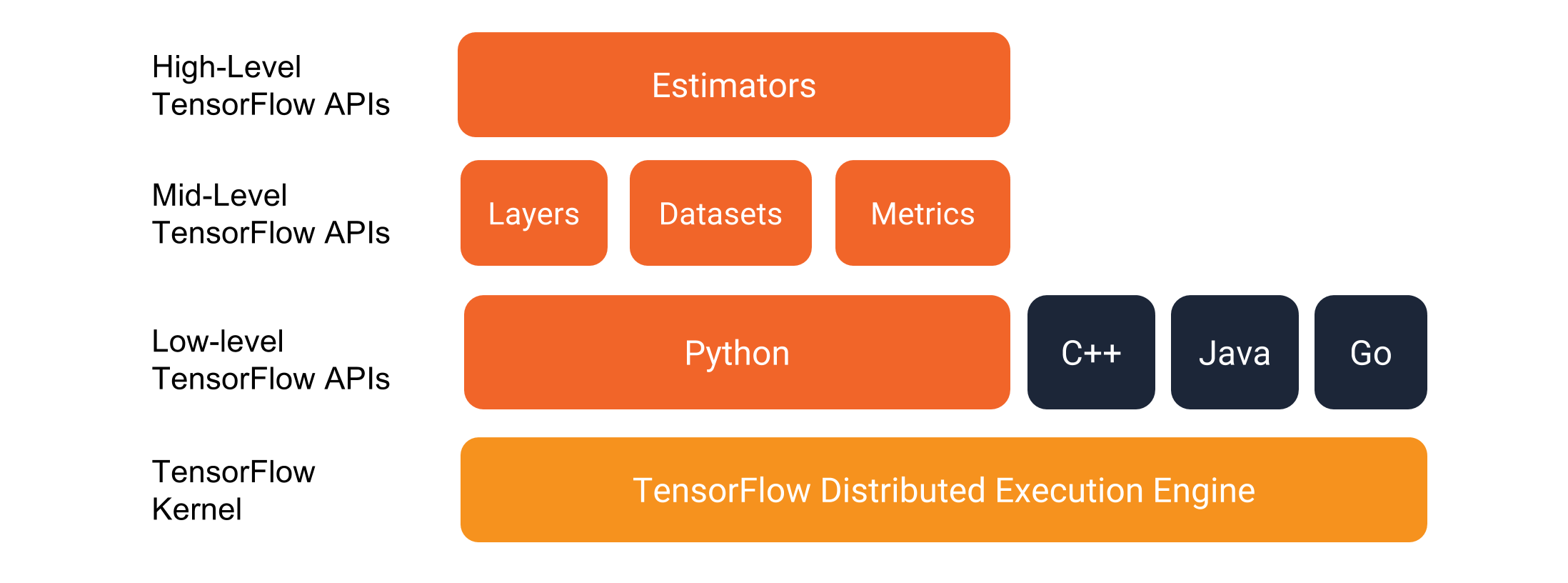Jarvis' Blog (总有美丽的风景让人流连) 总有美丽的风景让人流连

# Tensorflow Estimator 详解

2019-07-19
Jarvis
Post

Tensorflow 中目前主推的科研向API为 `tf.keras`, 而另一个封装性更高的高阶API `Estimator` 集成了常用的深度学习模型的操作:

• 训练
• 评估
• 预测
• 导出以供使用## 1. Estimator 基本结构

Estimator 本质上就是一个管理员, 他同时负责了模型的训练, 评估, 预测和导出.

### 1.1 预创建的 Estimator

Tensorflow 官方提供了一些简单的预创建 Estimator 以供直接使用:

• `tf.estimator.BaselineClassifier`
• `tf.estimator.BaselineRegressor`
• `tf.estimator.DNNLinearCombinedClassifier`
• `tf.estimator.DNNLinearCombinedRegressor`
• `tf.estimator.LinearClassifier`
• `tf.estimator.LinearRegressor`

### 1.2 自定义的 Estimator

Estimator 类创建的签名如下:

``````1
2
3
4
5
6
7
8
class EstimatorV2(object):
def __init__(self,
model_fn,
model_dir=None,
config=None,
params=None,
warm_start_from=None):
pass
``````

``````1
2
3
4
5
6
7
8
# 创建 Estimator
esti = tf.estimator.Estimator(model_fn, model_dir, config, params)
# 训练
esti.train(input_fn, hooks, steps, max_steps, saving_listeners)
# 验证
esti.evaluate(input_fn, steps, hooks, checkpoint_path, name)
# 预测
esti.predict(input_fn, predict_keys, hooks, checkpoint_path, yield_single_examples)
``````

1. 模型函数 `model_fn`
2. 数据输入函数 `input_fn`
3. 定义所有传入 Estimator 的参数: `config`, `params` 及其他参数
4. 钩子 `hooks`

### 1.3 模型函数

``````1
2
def model_fn(features, labels, mode, params, config):
pass
``````

``````1
2
def model_fn(features, labels, mode, params):
pass
``````

Estimator 的训练流程(`session.run()`)是封装在类内部的, 同时没有提供 `feed_dict` 的参数结构, 因此我们不能像 Tensorflow 低阶API中那样使用 `placeholder` 馈送数据到模型中. 官方建议的方式是用 `tf.data.Dataset` 类创建数据输入管线, 其用法也相当灵活, 可以参考 Tensorflow 数据输入管线, 这里就不再赘述. 其最终目标就是数据输入函数 `input_fn` 返回的必须是 `tf.Tensor` 或其嵌套(nested)对象, 从而 `model_fn` 接收到的也是 Tensor.

• `tf.estimator.ModeKeys.TRAIN`
• `tf.estimator.ModeKeys.EVAL`
• `tf.estimator.ModeKeys.PREDICT`

``````1
2
3
4
5
6
7
8
9
10
11
12
13
14
15
16
17
18
19
class EstimatorSpec(
collections.namedtuple('EstimatorSpec', [
'mode', 'predictions', 'loss', 'train_op', 'eval_metric_ops',
'export_outputs', 'training_chief_hooks', 'training_hooks', 'scaffold',
'evaluation_hooks', 'prediction_hooks'
])):
def __new__(cls,
mode,
predictions=None,
loss=None,
train_op=None,
eval_metric_ops=None,
export_outputs=None,
training_chief_hooks=None,
training_hooks=None,
scaffold=None,
evaluation_hooks=None,
prediction_hooks=None):
pass
``````

``````1
2
3
4
5
6
7
8
9
10
11
12
13
14
15
16
17
18
19
20
21
22
# Construct networks...

if mode == tf.estimator.ModeKeys.TRAIN:
# ...code...
return tf.estimator.EstimatorSpec(mode=mode, loss=loss, train_op=train_op)

if mode == tf.estimator.ModeKeys.EVAL:
# ...code...
metrics = {'accuracy': accuracy}
tf.summary.scalar('accuracy', accuracy)
return tf.estimator.EstimatorSpec(mode=mode, loss=loss, eval_metric_ops=metrics)

if mode == tf.estimator.ModeKeys.PREDICT:
# ...code...
predicted_classes = tf.argmax(logits, 1)
predictions = {
'class_ids': predicted_classes[:, tf.newaxis],
'probabilities': tf.nn.softmax(logits),
'logits': logits,
}
return tf.estimator.EstimatorSpec(mode=mode, predictions=predictions)
``````

### 1.4 数据输入函数

``````1
2
3
4
5
6
7
# 仅从 Estimator 传入 mode 参数
def input_fn(args1, args2, kwargs1, kwargs2, mode):
pass

# 从 Estimator 传入 mode, params 和 config 参数
def input_fn(args1, args2, kwargs1, kwargs2, mode, params, config):
pass
``````

### 1.5 Estimator 参数

Estimator 中关键的参数主要是 `params``config`.

#### 1.5.1 `config` 参数

`config` 参数是一个 `tf.estimator.RunConfig` 实例, 包含了配置设备, 训练流程控制, 分布式等参数. 类的签名如下:

``````1
2
3
4
5
6
7
8
9
10
11
12
13
14
15
16
17
18
19
class RunConfig(object):
"""This class specifies the configurations for an `Estimator` run."""
def __init__(self,
model_dir=None,
tf_random_seed=None,
save_summary_steps=100,
save_checkpoints_steps=_USE_DEFAULT,
save_checkpoints_secs=_USE_DEFAULT,
session_config=None,
keep_checkpoint_max=5,
keep_checkpoint_every_n_hours=10000,
log_step_count_steps=100,
train_distribute=None,
device_fn=None,
protocol=None,
eval_distribute=None,
experimental_distribute=None,
experimental_max_worker_delay_secs=None):
pass
``````

• `model_dir` 参数和 Estimator 中的同名参数相同, 两种方式都可以提供, 同时提供必须保证相同. 该参数指定了summary和模型保存的文件夹.
• `session_config` 是一个 `tf.ConfigProto`实例, 用于提供硬件设备配置, 如显存使用率, 显存是否按需增长, 是否允许变量所在设备的妥协(没有GPU时放在CPU上), CPU的使用数量, 操作内并行和操作间并行的线程数量等等.
• `train_distribute` 用来指定分布式训练策略, 如 `tf.distribute.MirroredStrategy`.

#### 1.5.2 `params` 参数

`params` 参数就是一个Python字典, 可以传入任何用户定义的参数, 从上面的讨论我们知道该参数可以传递到 `model_fn``input_fn`, 为用户参数传递提供了方便.

#### 1.5.3 `warm_start_from` 参数

• 该参数可以传入一个Tensorflow检查点, 此时会把计算图中所有的可训练参数从检查点中初始化.
• 该参数也可以传入一个 `tf.estimator.WarmStartSettings` 的实例, 该类也是一个属性类, 提供了参数可以指定需要从检查点初始化的变量, 从而避免初始化所有变量.

Estimator 是使用 `tf.train.init_from_checkpoint()` 载入检查点的.

(未完待续)

Content